NCERT Solutions for Class 7 Maths Chapter 11 Perimeter and Area Ex 11.2 are part of NCERT Solutions for Class 7 Maths. Here we have given NCERT Solutions for Class 7 Maths Chapter 11 Perimeter and Area Ex 11.2.

 Board CBSE Textbook NCERT Class Class 7 Subject Maths Chapter Chapter 11 Chapter Name Perimeter and Area Exercise Ex 11.2 Number of Questions Solved 8 Category NCERT Solutions

## NCERT Solutions for Class 7 Maths Chapter 11 Perimeter and Area Ex 11.2

Question 1.
Find the area of the following parallelograms: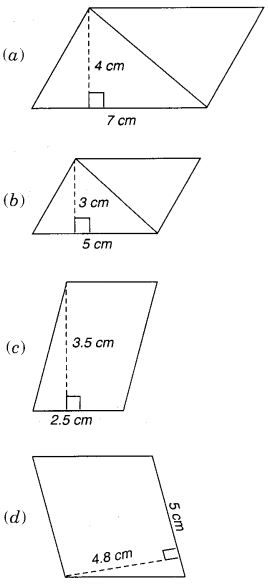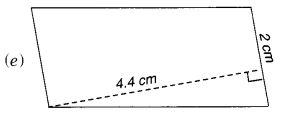Solution: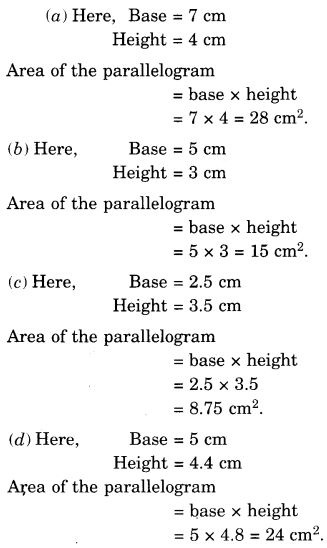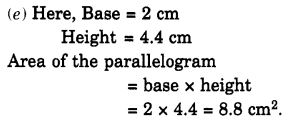Question 2.
Find the area of each of the following triangles: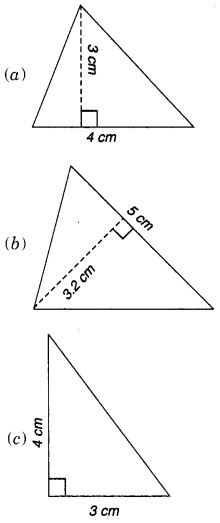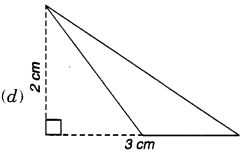Solution: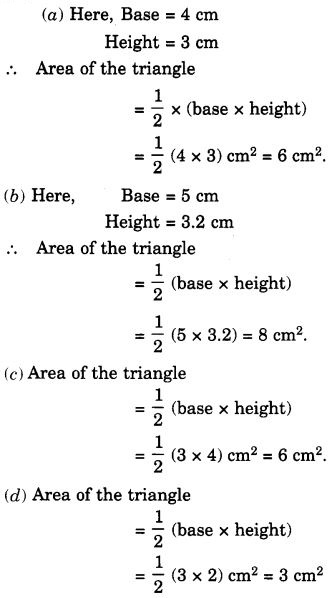Question 3.
Find the missing values: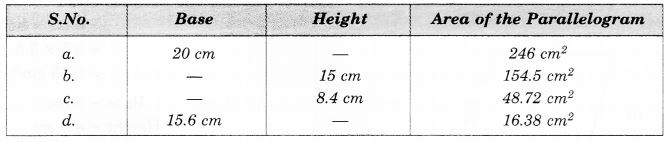Solution:Question 4.
Find the missing values: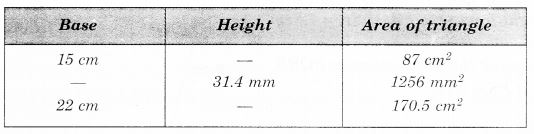Solution: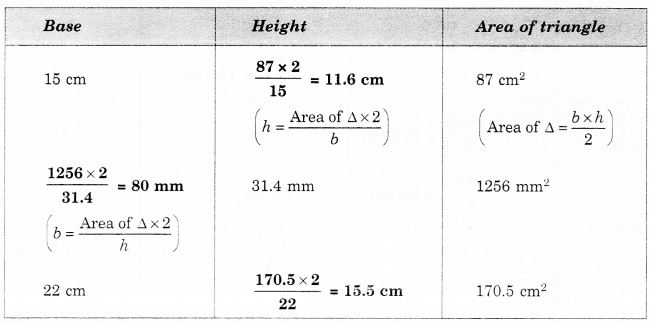Question 5.
PQRS is a parallelogram (in Figure). QM is the height from Q to SR and QN is the height from Q to PS. If SR = 12 cm and QM = 7.6 cm. Find:
(a) the area of the parallelogram PQRS
(b) QN, if PS = 8 cm.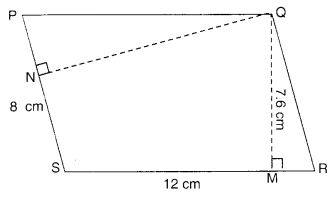Solution:
(a) Area of the parallelogram PQRS = base × height = SR × QM = 12 × 7.6 cm2 = 91.2 cm2
(b) Area of the parallelogram PQRS = base × height = PS × QN
⇒ 91.2 = 8 × QN
⇒ QN = $$\frac { 912 }{ 8 }$$ cm
⇒ QN = 11.4 cm.

Question 6.
DL and BM are the heights on sides AB and AD respectively of parallelogram ABCD (in Figure). If the area of a parallelogram is 1470 cm2, AB = 35 cm, and AD = 49 cm, find the length of BM and DL.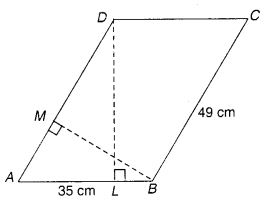Solution: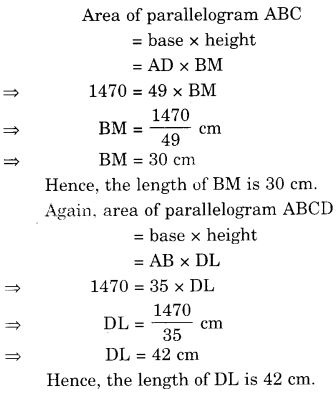Question 7.
∆ ABC is right-angled at A (in Figure). AD is perpendicular to BC. If AB = 5 cm, BC = 13 cm and AC = 12 cm, find the area of ∆ ABC. Also, find the length of AD.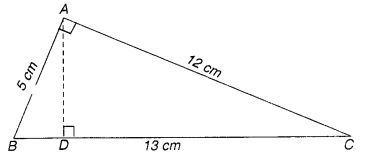Solution: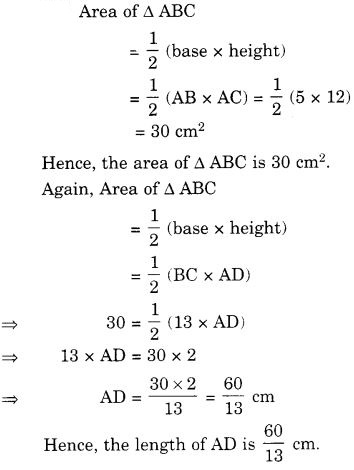Question 8.
∆ ABC is isosceles with AB = AC = 7.5 cm, and BC = 9 cm (in Figure). The height of AD from A to BC is 6 cm. Find the area of ∆ ABC. What will be the height from C to AB i.e., CE?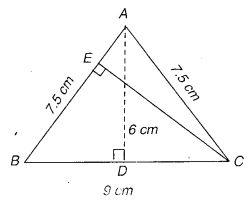Solution: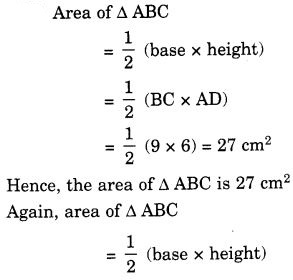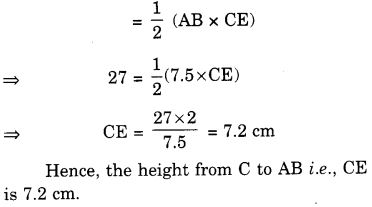We hope the NCERT Solutions for Class 7 Maths Chapter 11 Perimeter and Area Ex 11.2 help you. If you have any query regarding NCERT Solutions for Class 7 Maths Chapter 11 Perimeter and Area Ex 11.2, drop a comment below and we will get back to you at the earliest.